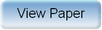Ninth International Geostatistics Congress, Oslo, Norway
June 11 – 15, 2012Session: Mining 4 Abstract No.: O-074 Title: Multidirectional Random Walk: An Alternative to Local Simulation of Gaussian Data Author(s): D. T. Ribeiro, Vale (BR)E. M. Cunha Filho, Vale (BR)J. F. C. L. Costa, UFRGS (BR)D. G. Roldao, Vale (BR) Abstract: Various geostatistical simulation techniques rely on the multigaussian model. These methods generate equally probable realizations of a gaussian variable, honoring the first two statistical moments, i.e. the histogram and the spatial variability function (h) of the gaussian variable. Currently existing techniques are time consuming to implement and require advanced computational hardware to process real dataset and millions of grid nodes. The study report an alternative simulation technique based on multidirectional random walk of gaussian variables. This method is based on random walks that consider the action of a particle in random directions along a real line. The position of the particle in a determined distance d is described by an independent random increment, each one with the same probability, of 50%, to be equal 1 or -1. The increments at a certain distance d have a normal distribution, with mean zero and variance proportional to the distance. The random walk simulation technique is based on conditioning points, generating an error Yrw as a function of distance d which should be added to the conditioning value Y(x) to obtain the simulated value Ysrw(x+d). Although, for cases of multidirectional random walk from more than one conditioning point, simple kriging weights must be used, which is given by the following equation: Ysrw(x0)=ini[Yxi+Yrw(dxix0)] Where: i is the simple kriging weight; x0 is the point to be simulated; xi is the conditioning point; d is the distance from x0 to xi; and n is the number of conditioning points. The experimental results shows this new methodology honored both two statistical moments of the gaussian conditioning points faster than sequential algorithms.Produced by X-CD Technologies Inc.